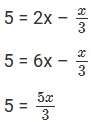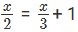# Chapter 8: Linear Equations in One Variable Exercise – 8.1

In this chapter, we provide RD Sharma Class 7 ex 8.1 Solutions Chapter 8 Linear Equations in One Variable for English medium students, Which will very helpful for every student in their exams. Students can download the latest RD Sharma Class 7 ex 8.1 Solutions Chapter 8 Linear Equations in One Variable Maths pdf, Now you will get step by step solution to each question.

## RD Sharma Solutions for Class 7 Chapter 8 Linear Equations in One VariableEx 8.1 Download PDF

### Question: 1

Verify by substitution that:

(i) x = 4 is the root of 3x – 5 = 7

(ii) x = 3 is the root of 5 + 3x = 14

(iii) x = 2 is the root of 3x – 2 = 8x – 12

(iv) x = 4 is the root of 3x/2 = 6

(v) y = 2 is the root of y – 3 = 2y – 5

(vi) x = 8 is the root of (1/2)x + 7 = 11

### Solution:

(i) x = 4 is the root of 3x – 5 = 7.

Now, substituting x = 4 in place of ‘x’ in the given equation 3x – 5 = 7,

3(4) – 5 = 7

12 – 5 = 7

7 = 7

Since, LHS = RHS

Hence, x = 4 is the root of 3x – 5 = 7.

(ii). x = 3 is the root of 5 + 3x = 14.

Now, substituting x = 3 in place of ‘x’ in the given equation 5 + 3x = 14,

5 + 3(3) = 14

5 + 9 = 14

14 = 14

Since, LHS = RHS

Hence, x = 3 is the root of 5 + 3x = 14.

(iii). x = 2 is the root of 3x – 2 = 8x – 12.

Now, substituting x = 2 in place of ‘x’ in the given equation 3x –2 = 8x – 12,

3(2) – 2 = 8(2) – 12

6 – 2 = 16 – 12

4 = 4

Since, LHS = RHS

Hence, x = 2 is the root of 3x – 2 = 8x – 12.

(iv) x = 4 is the root of 3x/2 = 6.

Now, substituting x = 4 in place of ‘x’ in the given equation 3x/2 = 6,

(3× 4)/2 = 6

12/2 = 6

6 = 6

Since, LHS = RHS

Hence, x = 4 is the root of 3x/2 = 6.

(v). y = 2 is the root of y – 3 = 2y – 5.

Now, substituting y = 2 in place of ‘y’ in the given equation y – 3 = 2y – 5,

2 – 3 = 2(2) – 5

-1 = 4 – 5

-1 = -1

Since, LHS = RHS

Hence, y = 2 is the root of y – 3 = 2y – 5.

(vi). x = 8 is the root of 12x + 7 = 11.

Now, substituting x = 8 in place of ‘x’ in the given equation 12x + 7 = 11,

12(8) + 7 =11

4 + 7 = 11

11 = 11

Since, LHS = RHS

Hence, x = 8 is the root of 12x + 7 = 11.

### Question: 2

Solve each of the following equations by trial and error method:

(i) x + 3 = 12

(ii) x – 7 = 10

(iii) 4x = 28

(iv) x/2 + 7 = 11

(v) 2x + 4 = 3x

(vi) x/4 = 12

(vii) 15/x = 3

(viii) x/18 = 20

### Solution:

(i) x + 3 = 12

Here, LHS = x + 3 and RHS = 12

Therefore, if x = 9, LHS = RHS.

Hence, x = 9 is the solution to this equation.

(ii) x – 7 = 10

Here, LHS = x – 7 and RHS = 10.

Therefore, if x = 17, LHS = RHS.

Hence, x = 17 is the solution to this equation.

(iii) 4x = 28

Here, LHS = 4x and RHS = 28.

Therefore, if x = 7, LHS = RHS

Hence, x = 7 is the solution to this equation.

(iv) x/2 + 7 = 11

Here, LHS = x/2 + 7 and RHS = 11.

Since RHS is a natural number, x/2 must also be a natural number, so we must substitute values of x that are multiples of 2.

Therefore, if x = 8, LHS = RHS.

Hence, x = 8 is the solution to this equation.

(v) 2x + 4 = 3x

Here, LHS = 2x + 4 and RHS = 3x.

Therefore, if x = 4, LHS = RHS.

Hence, x = 4 is the solution to this equation.

(vi) x/4 = 12

Here, LHS = x/4 and RHS = 12.

Since RHS is a natural number, x/4 must also be a natural number, so we must substitute values of x that are multiples of 4.

Therefore, if x = 48, LHS = RHS.

Hence, x = 48 is the solution to this equation.

(vii) 15x = 3All Chapter RD Sharma Solutions For Class 7 Maths

I think you got complete solutions for this chapter. If You have any queries regarding this chapter, please comment on the below section our subject teacher will answer you. We tried our best to give complete solutions so you got good marks in your exam.